# Equivalent Fractions: Missing Numerator or Denominator

Equivalent Fractions: Missing Numerator or Denominator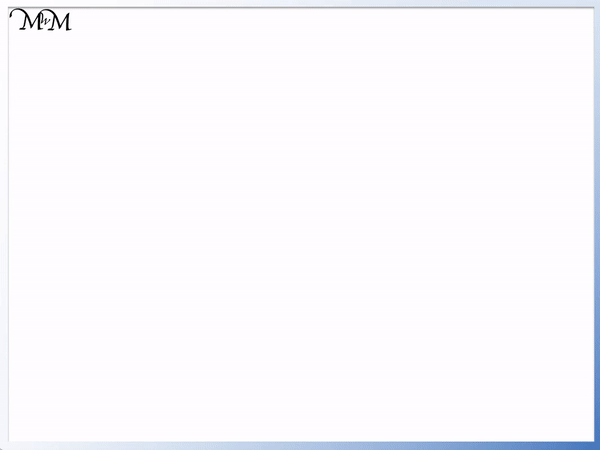• Equivalent fractions are two fractions that are written differently but have the same size.
• The two fractions:   1 / 3   and   2 / 6 are equivalent fractions.
• We can multiply the of '1' by two to get a new numerator of '2'.
• Because we multiplied the numerator by two, we multiply the by two as well.
• 3 x 2 = 6.
• We can also go from   2 / 6   to   1 / 3   by dividing.
• 2 ÷ 2 = 1 and 6 ÷ 2 = 3.
To find an equivalent fraction, multiply or divide the numerators and denominators by the same number.• The equals sign between the two fractions tells us that they equivalent.
• Since we have equivalent fractions, we must multiply or divide by the same number on the top as we do on the bottom.
• The denominator of 4 has been multiplied by 8 to get the new denominator of 32.
• To find our missing numerator, we multiply the known numerator by 8 as well.
• 3 x 8 = 24.
• In the next example, our numerator of 4 has been divided by 4 to make 1.
• So to find our missing denominator, we also divide the known denominator by 4.
• 20 ÷ 4 = 5.# Finding the Missing Numerator or Denominator

## How to find the Numerator and Denominator of a Fraction

The numerator is the number on top of the fraction, above the dividing line. The denominator is the number on the bottom of the fraction, below the dividing line.

To find a numerator, read the number on the top of the fraction.

To find a denominator, read the number on the bottom of the fraction.

For example in the fraction   1 / 2   , the numerator is 1 and the denominator is 2.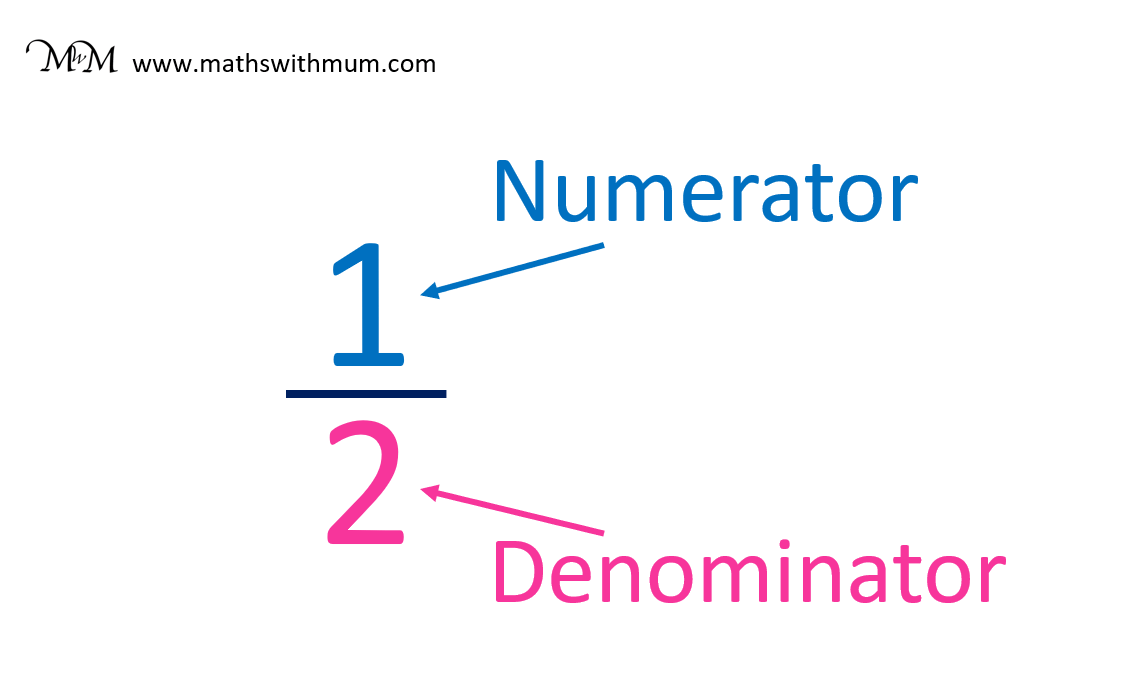We can find a missing numerator or denominator in an equivalent fraction.

An equivalent fraction is made by multiplying or dividing the numerator and denominator of a fraction by the same amount.

For example,   1 / 3   is equivalent to   2 / 6  .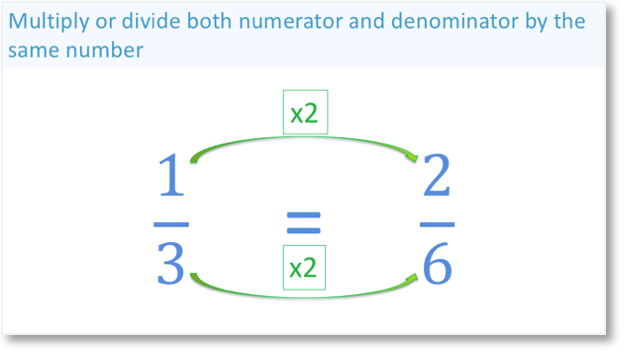To create an equivalent fraction multiply the numerator and denominator by the same amount.

In this example, we have multiplied the numerator by 2 and the denominator by 2.

When first teaching finding missing numbers in fractions, it is often helpful to include the arrows from each numerator and denominator as shown in the image above.

This method helps children to stick carefully to the idea that we are multiplying or dividing by the same values on the top and on the bottom of our fraction.

Here is the same process for filling in a blank of a fraction shown in reverse. Dividing the numerator and denominator by the same amount will make an equivalent fraction.We have divided 6 and 2 in half.

## How to Find the Missing Numerator of a Fraction

To find a missing numerator, follow these steps:

1. Find the amount that the first denominator is multiplied or divided by to get to the denominator that is missing its numerator.
2. Multiply or divide the known numerator by this same amount to find the unknown numerator.

Here is an example of finding a missing numerator. We have   3 / 4   =   / 32  .

We need to fill in the blank for this fraction.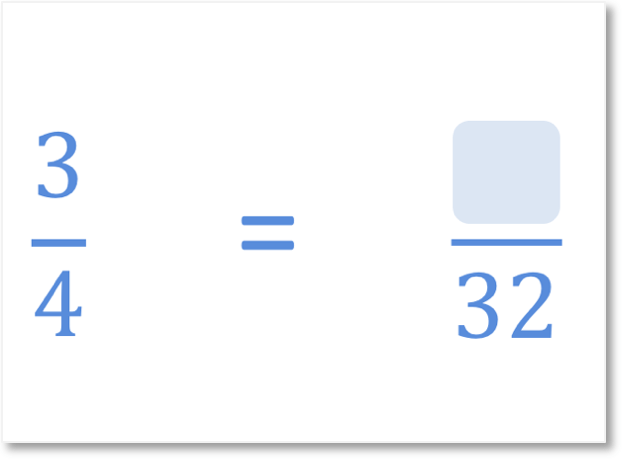In simple terms, use the following steps to find an unknown numerator:

• Step 1: What do we multiply the denominators by?
• Step 2: Multiply the known numerator by this same value to find the missing numerator.

We have multiplied 4 by 8 to get 32.

To find the amount that one denominator has been multiplied by in an equivalent fraction, divide the largest denominator by the smallest denominator.

32 ÷ 4 = 8 and so, the numerator and denominator in this equivalent fraction have been multiplied by 8.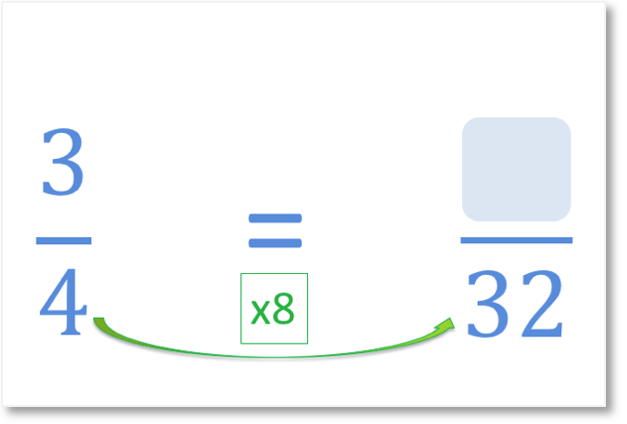We multiply 3 by 8 to get 24.

The missing numerator in this question is 24.To find a missing numerator, look at the denominators of the fractions.

One fraction has both a numerator and denominator. Find the number that this denominator is multiplied by to get to the denominator that is missing its numerator.

Multiply the known numerator by this number to find the unknown numerator.

## How to Find the Missing Denominator of a Fraction

To find a missing denominator, follow these steps:

1. Find the amount that the first numerator is multiplied or divided by to get to the numerator that is missing its denominator.
2. Multiply or divide the known denominator by this same amount to find the unknown denominator.

For example, here we have a question with a fraction missing its denominator.   1 / 3   =   3 /  .

We need to fill in the blank for this fraction.In simple terms, use the following steps to find an unknown denominator:

• Step 1: What do we multiply the numerators by?
• Step 2: Multiply the known denominator by this same value to find the missing denominator.

We have multipled the first numerator by 3.

To find the value that a numerator has been multiplied by in an equivalent fraction, divide the largest numerator by the smallest numerator.

9 ÷ 3 = 3 and so, the first fraction has been multiplied by 3.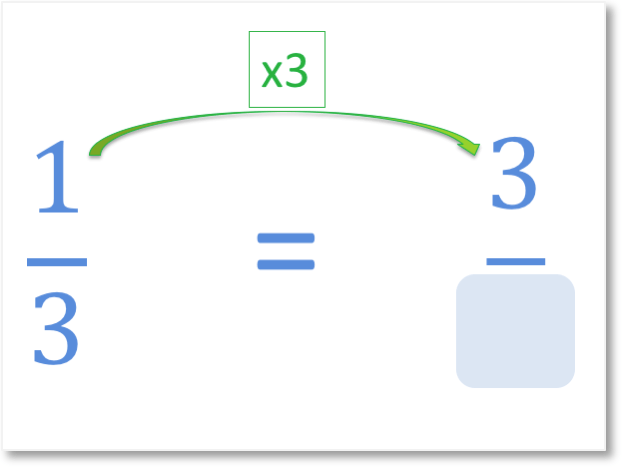The next step is to multiply the known denominator by 3 to find the unknown denominator.

3 multiplied by 3 is 9 and so, the missing denominator is 9.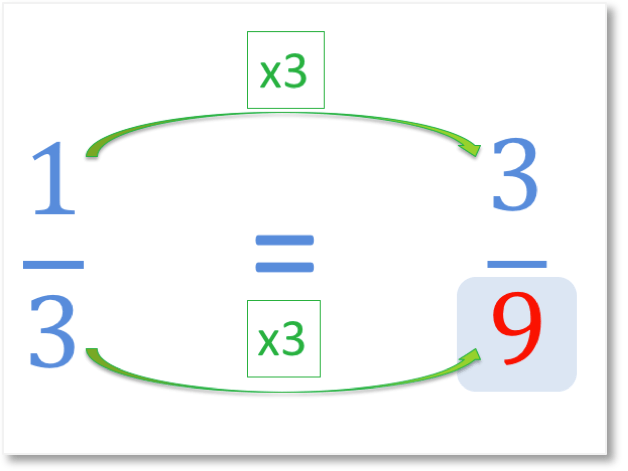To find the missing denominator, first look at the numerators of the fractions.

One fraction has both a numerator and denominator. Find the number that this numerator is multiplied by to get to the numerator that is missing its denominator.

Multiply the known denominator by this number to find the unknown denominator.

Here is another example of finding a missing denominator. In this question, we are dividing to find the unknown denominator.

We have   4 / 20   =   1 /  .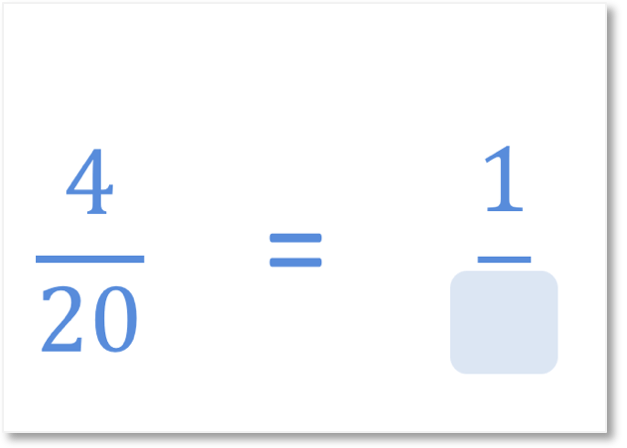We can see that the 4 has been divided by 4 to get a numerator of 1 in the fraction on the right.Since the numerator of 4 has been divided by 4, the denominator of 20 is also divided by 4.

20 ÷ 4 = 5 and so, the unknown denominator is 5.The missing denominator is 5.

When teaching filling in the blanks of equivalent fractions to your child, the arrows can be useful for introducing the procedure and idea of equivalency, however it is normal that with practice that they will be able to do these questions without using the arrows.

The understanding of this procedure can be impeded by slow times table recall and I would recommend a times table warm up first using our Multiplication Grid. Then follow up this topic by practising with the 'Equivalent Fractions: Finding a Missing Numerator or Denominator' worksheets above.Now try our lesson on Simplifying Fractions where we learn how to use our knowledge of equivalent fractions in to simplify a fraction.